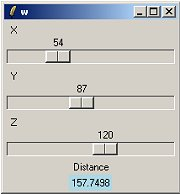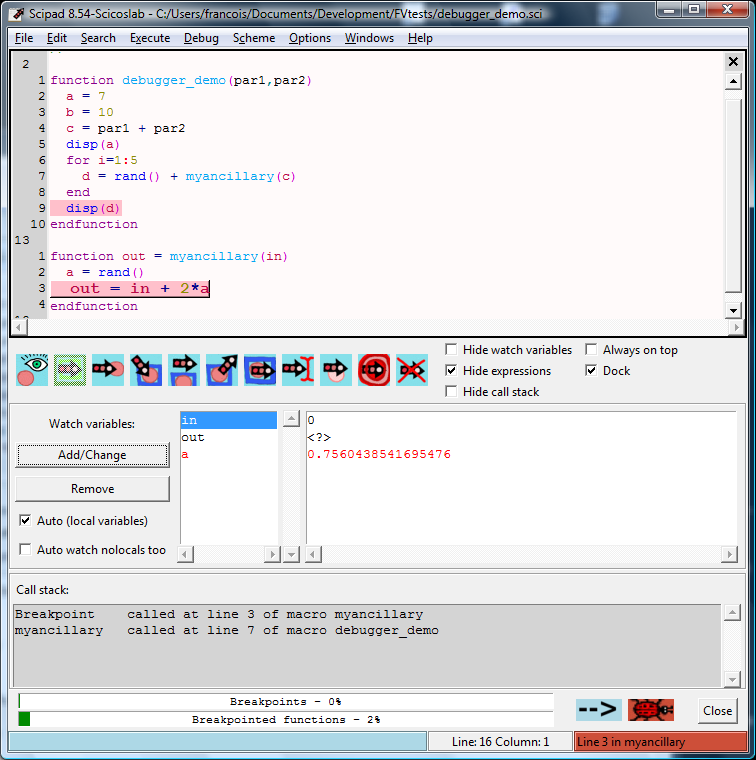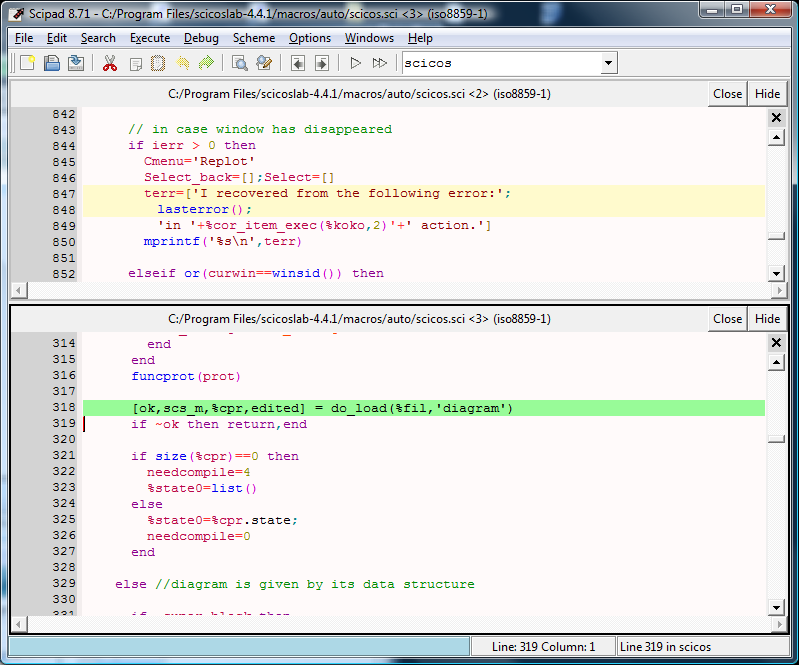## SciLabScilab is a free program for numerical computation. It is used for numerical computations in engineering, statistics and for plotting graphs and such.

It has connection to Tcl via these functions:

ScilabEval
Tcl instruction: Evaluate a string with scilab interpreter
TK_EvalFile (obsolete, replaced by TCL_EvalFile)
Reads and evaluate a tcl/tk file
TK_EvalStr (obsolete, replaced by TCL_EvalStr)
Evaluate a string whithin the tcl/tk interpreter
TK_GetVar (obsolete, replaced by TCL_GetVar)
Get a tcl/tk variable value
TK_SetVar (obsolete, replaced by TCL_SetVar)
Set a tcl/tk variable value
close
close a figure
figure
create a figure
findobj
find an object with specified property
gcf
gets the current active tksci figure
uicontrol
create a Graphic User Interface object

Scilab's help system is implemented using Tk.

GS 2012-06-11: Here is a simple example for using Tcl-Tk with Scilab. There is no tclsh or wish in Scilab. Scilab evals commands with the ScilabEval function.There are 3 files (run.sce, distance.sci, gui.tcl).

The Scilab function TCL_EvalFile is used to display the GUI, which calls a Scilab function to recompute the distance (function distance()) each time sliders are moved.

To make a test, put these 3 files in the same directory, run Scilab and change the working directory:

`cd <your Scilab directory>`

Enter the command:

`exec ('run.sce')`

and the GUI will display.

The file run.sce:

```// run.sce
exec distance.sci;
TCL_EvalFile('gui.tcl');```

the file distance.sci:

```// distance.sci
function dist = distance(x,y,z)
dist = sqrt(x*x + y*y + z*z)
endfunction```

And the Tcl-Tk code:

```# gui.tcl
global x y z dist

set x 10
set y 10
set z 10
set dist { }

toplevel .w
scale .w.scx -from 0 -to 200 -length 200 -resolution 1 -label X \
-orient horiz -bd 1 -showvalue true -variable x \
-command ComputeDistance
scale .w.scy -from 0 -to 200 -length 200 -resolution 1 -label Y \
-orient horiz -bd 1 -showvalue true -variable y \
-command ComputeDistance
scale .w.scz -from 0 -to 200 -length 200 -resolution 1 -label Z \
-orient horiz -bd 1 -showvalue true -variable z \
-command ComputeDistance

label .w.lt -text Distance
label .w.ldist -textvariable dist -bg lightblue
eval pack [winfo children .w]

proc ComputeDistance w {
global x y z dist
ScilabEval "dist = distance(\$x,\$y,\$z);TK_SetVar('dist',string(dist));"
}```

Scipad%|%Scipad is a powerful editor and graphical debugger for programs written in the Scilab language. It is a mature and highly configurable editor, almost entirely written in Tcl/Tk.